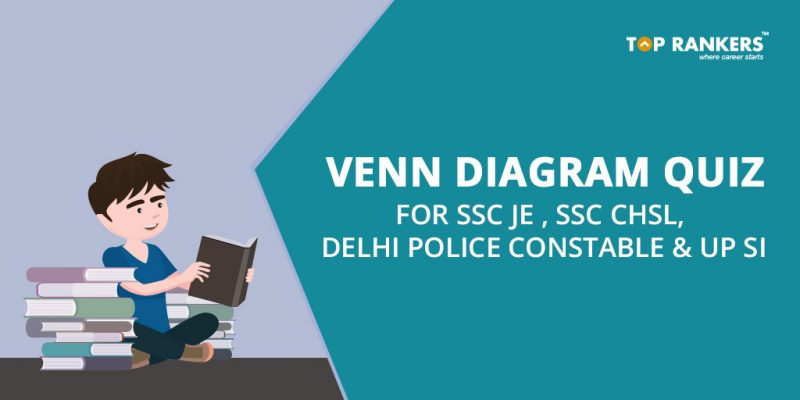# Venn Diagram Quiz for SSC JE , SSC CHSL, Delhi Police Constable and UP SIVenn Diagram Quiz for SSC JE , SSC CHSL, Delhi Police Constable and UP SI- Solve venn diagram quiz questions with memory based questions for SSC JE , SSC CHSL, Delhi Police Constable and UP SI

## Venn Diagram Quiz for SSC JE , SSC CHSL, Delhi Police Constable and UP SI

Venn Diagram Quiz for SSC JE , SSC CHSL, Delhi Police Constable and UP SI- Venn diagram is a topic asked in verbal reasoning section in competitive exams. Venn diagram is definitely not a new topic for us as we have already solved in our school days. Being one of the trickiest of all, the topic demands you to think critically. You need to analyze and have strong logical skills to have a command over this topic. Healthy Practice will help you gain an expertise in this topic. Toprankers brings you Venn Diagram Quiz for SSC JE , SSC CHSL, Delhi Poilice Constable and UP SI, a part of the FREE daily quiz initiative. This has been introduced to help you overcome your flaws and perform well in this topic.

Take QUIZ Here

### Where to attempt Venn Diagram Quiz for SSC JE , SSC CHSL, Delhi Police Constable and UP SI?

You need to follow the steps given below:

Step 1- Click on the link provided in this post or visit- https://www.toprankers.com/ssc/ssc-daily-quiz on 29th December 2017.

Step 2 – You will have to register in case you are not. But, if you are registered, you can directly log in using your credentials.

Step 3 – You will see a section named “General Knowledge Economics Quiz”. In order to take the test, click on “Take test”.

Step 4 – Go through the instructions and get started with the quiz.

### Tips to Solve Venn Diagram Quiz for SSC JE , SSC CHSL, Delhi Police Constable and UP SI

A student has to look at the diagram and solve as per instructions. The most crucial step is to pay attention to the details.

Let’s take a look at some basic formulas for Venn diagrams of 2 and 3 elements:

• n ( A ∪ B) = n(A ) + n ( B ) – n ( A∩ B)
• n (A ∪ B ∪ C) = n(A ) + n ( B ) + n (C) – n ( A ∩ B) – n ( B ∩ C) – n ( C ∩ A) + n (A ∩ B ∩ C )

where n( A) = number of elements in set A.
Once you get well- versed with concepts of Venn diagram with the help of diagrams, you won’t have to remember/ memorize any formula.

### Venn Diagram for two elementsWhere;
X = number of elements that belong to set A only
Y = number of elements that belong to set B only
Z = number of elements that belong to set A and B both (AB)
W = number of elements that belong to none of the sets A or B
From the above figure, it is clear that
n(A) = x + z ;
n (B) = y + z ;
n(A ∩ B) = z;
n ( A ∪ B) = x +y+ z.
Total number of elements = x + y + z + w

### Venn Diagram for three elementsWhere,
W = number of elements that belong to none of the sets A, B or C

Please note that you must always start filling values in the Venn diagram from the innermost value.

### Venn Diagram Quiz for SSC JE , SSC CHSL, Delhi Police Constable and UP SI- Benefits

• You get to discover which areas you are lagging in
• You practice questions as per latest pattern
• Your confidence level is boosted

FREE Quizzes for banking

Are you interested for more? We will keep posting about FREE quizzes on various topics in our FREE Daily Quiz Section. Stay tuned!

Keep visiting us for latest updates on Venn Diagram Quiz for SSC JE , SSC CHSL, Delhi Police Constable and UP SI.

All the best!